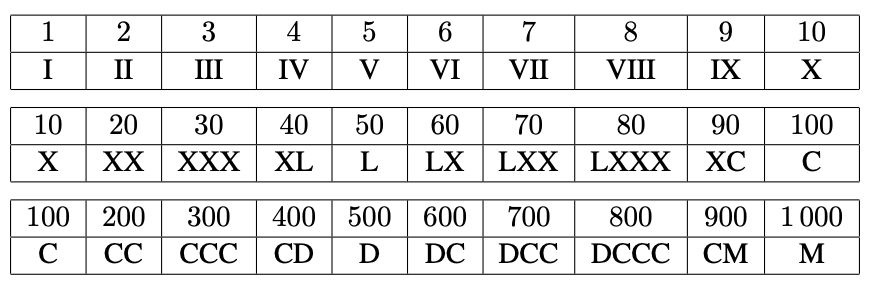시간 제한 메모리 제한 제출 정답 맞은 사람 정답 비율
2 초 512 MB 0 0 0 0.000%

## 문제

The ancient Romans created many important things: aqueducts, really straight roads, togas, those candles that spout fireworks. But the most useless is Roman numerals, a very awkward way to represent positive integers.

The Roman numeral system uses seven different letters, each representing a different numerical value: the letter I represents the value 1, V 5, X 10, L 50, C 100, D 500 and M 1 000. These can be combined to form the following base values:The Roman numeral representation of a non-base value number x is obtained by first breaking up x into a sum of base values and then translating each base value, largest to smallest. When choosing base values you always choose the largest one ≤ x first, then the largest one ≤ the amount remaining, and so on. Thus 14 = 10 + 4 = XIV, 792 = 700 + 90 + 2 = DCCXCII. Numbers larger than 1 000 use as many M’s as necessary. So 2 018 = MMXVIII and 1 000 000 would be a string of one thousand M’s (hence the word “awkward” in the first paragraph).

The Roman numeral representation gives a new way to order the positive integers. We can now order them alphabetically if we treat the Roman representation of each integer as a word. If one word A is a prefix for another word B then A comes first. We’ll call this the roman ordering of the positive integers. Thus the first number in roman ordering is C (100 in our system). The next three numbers would be CC, CCC and CCCI, and so on.

Note in roman ordering, all numbers larger than 1 000 would come before any number starting with V or X. Indeed the last number is XXXVIII. In this problem you will be given one or more positive integers and must determine their positions in the roman ordering – from the front or back as appropriate.

## 입력

Input starts with a positive integer n ≤ 100 indicating the number of positive integers to follow, each on a separate line. Each of these remaining numbers will be ≤ 109.

## 출력

For each value (other than n), output the position of the integer in the roman ordering, one per line. If the position is relative to the end of the roman ordering, make the integer negative. Thus 38 has roman ordering position −1, 37 has position −2, and so on.

## 예제 입력 1

3
100
101
38


## 예제 출력 1

1
302
-1